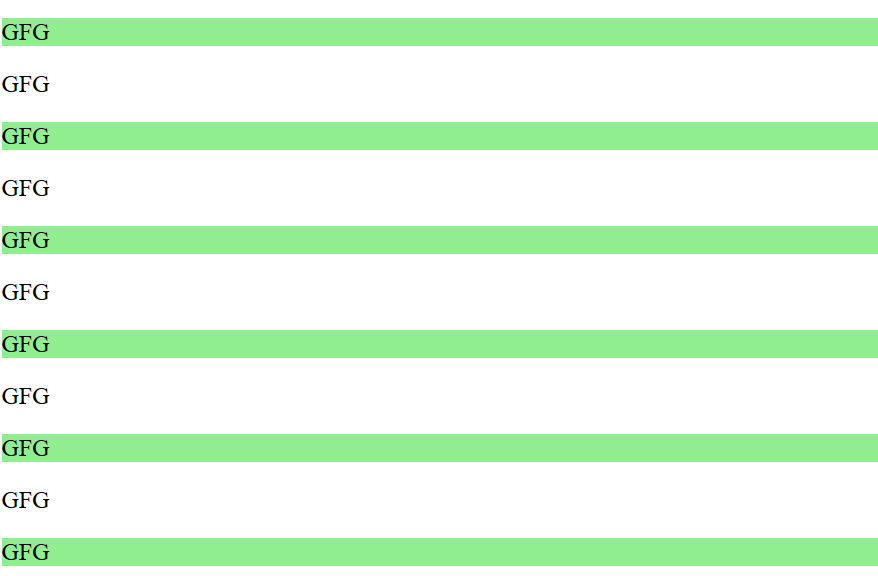Open in App
Not now

# jQuery :nth-child() Selector

• Last Updated : 14 Nov, 2022

The jQuery :nth-child() Selector Selects all elements that are the nth-child of their parent elements.

Syntax:

`\$("Selector:nth-child( index | even |odd |equation )")`
1. Index: Index provided. Index starts from
2. even: even number of child elements get selected.
3. odd: odd number of child elements will get selected.
4. equation: select child elements by solving a formula(an+b).

Example:

## HTML

 `` `<``html``>` `<``head``>` `  ``<``script` `src``=` `"https://ajax.googleapis.com/ajax/libs/jquery/3.3.1/jquery.min.js"``>` `  ``` `  ``<``script``>` `    ``\$(document).ready(function () {` `      ``\$("p:nth-child(2n-1)").css("background-color",` `        ``"lightgreen");` `    ``});` `  ``` `` `<``body``>` `  ``<``p``>GFG` `  ``<``p``>GFG` `  ``<``p``>GFG` `  ``<``p``>GFG` `  ``<``p``>GFG` `  ``<``p``>GFG` `  ``<``p``>GFG` `  ``<``p``>GFG` `  ``<``p``>GFG` `  ``<``p``>GFG` `  ``<``p``>GFG` `` ``

Output:My Personal Notes arrow_drop_up
Related Articles